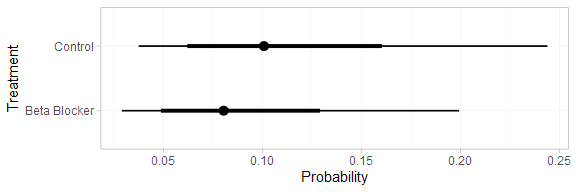# Example: Beta blockers

library(multinma)
options(mc.cores = parallel::detectCores())

This vignette describes the analysis of 22 trials comparing beta blockers to control for preventing mortality after myocardial infarction (Carlin 1992; Dias et al. 2011). The data are available in this package as blocker:

head(blocker)
#>   studyn trtn         trtc  r   n
#> 1      1    1      Control  3  39
#> 2      1    2 Beta Blocker  3  38
#> 3      2    1      Control 14 116
#> 4      2    2 Beta Blocker  7 114
#> 5      3    1      Control 11  93
#> 6      3    2 Beta Blocker  5  69

### Setting up the network

We begin by setting up the network - here just a pairwise meta-analysis. We have arm-level count data giving the number of deaths (r) out of the total (n) in each arm, so we use the function set_agd_arm(). We set “Control” as the reference treatment.

blocker_net <- set_agd_arm(blocker,
study = studyn,
trt = trtc,
r = r,
n = n,
trt_ref = "Control")
blocker_net
#> A network with 22 AgD studies (arm-based).
#>
#> ------------------------------------------------------- AgD studies (arm-based) ----
#>  Study Treatments
#>  1     2: Control | Beta Blocker
#>  2     2: Control | Beta Blocker
#>  3     2: Control | Beta Blocker
#>  4     2: Control | Beta Blocker
#>  5     2: Control | Beta Blocker
#>  6     2: Control | Beta Blocker
#>  7     2: Control | Beta Blocker
#>  8     2: Control | Beta Blocker
#>  9     2: Control | Beta Blocker
#>  10    2: Control | Beta Blocker
#>  ... plus 12 more studies
#>
#>  Outcome type: count
#> ------------------------------------------------------------------------------------
#> Total number of treatments: 2
#> Total number of studies: 22
#> Reference treatment is: Control
#> Network is connected

### Meta-analysis models

We fit both fixed effect (FE) and random effects (RE) models.

#### Fixed effect meta-analysis

First, we fit a fixed effect model using the nma() function with trt_effects = "fixed". We use $$\mathrm{N}(0, 100^2)$$ prior distributions for the treatment effects $$d_k$$ and study-specific intercepts $$\mu_j$$. We can examine the range of parameter values implied by these prior distributions with the summary() method:

summary(normal(scale = 100))
#> A Normal prior distribution: location = 0, scale = 100.
#> 50% of the prior density lies between -67.45 and 67.45.
#> 95% of the prior density lies between -196 and 196.

The model is fitted using the nma() function. By default, this will use a Binomial likelihood and a logit link function, auto-detected from the data.

blocker_fit_FE <- nma(blocker_net,
trt_effects = "fixed",
prior_intercept = normal(scale = 100),
prior_trt = normal(scale = 100))

Basic parameter summaries are given by the print() method:

blocker_fit_FE
#> A fixed effects NMA with a binomial likelihood (logit link).
#> Inference for Stan model: binomial_1par.
#> 4 chains, each with iter=2000; warmup=1000; thin=1;
#> post-warmup draws per chain=1000, total post-warmup draws=4000.
#>
#>                     mean se_mean   sd     2.5%      25%      50%      75%    97.5% n_eff Rhat
#> d[Beta Blocker]    -0.26    0.00 0.05    -0.36    -0.29    -0.26    -0.22    -0.16  3226    1
#> lp__            -5960.31    0.08 3.35 -5967.90 -5962.36 -5959.95 -5957.96 -5954.66  1852    1
#>
#> Samples were drawn using NUTS(diag_e) at Wed Nov 25 17:56:23 2020.
#> For each parameter, n_eff is a crude measure of effective sample size,
#> and Rhat is the potential scale reduction factor on split chains (at
#> convergence, Rhat=1).

By default, summaries of the study-specific intercepts $$\mu_j$$ are hidden, but could be examined by changing the pars argument:

# Not run
print(blocker_fit_FE, pars = c("d", "mu"))

The prior and posterior distributions can be compared visually using the plot_prior_posterior() function:

plot_prior_posterior(blocker_fit_FE, prior = "trt")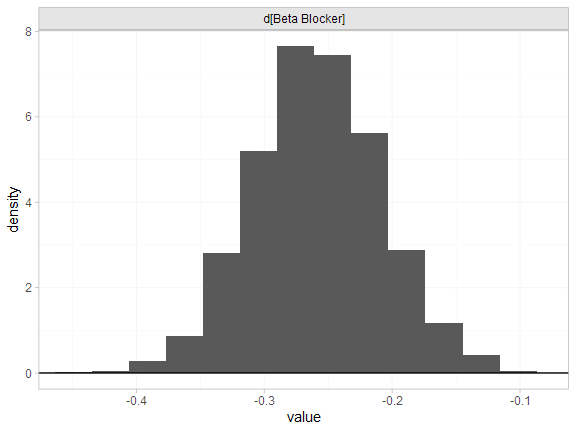#### Random effects meta-analysis

We now fit a random effects model using the nma() function with trt_effects = "random". Again, we use $$\mathrm{N}(0, 100^2)$$ prior distributions for the treatment effects $$d_k$$ and study-specific intercepts $$\mu_j$$, and we additionally use a $$\textrm{half-N}(5^2)$$ prior for the heterogeneity standard deviation $$\tau$$. We can examine the range of parameter values implied by these prior distributions with the summary() method:

summary(normal(scale = 100))
#> A Normal prior distribution: location = 0, scale = 100.
#> 50% of the prior density lies between -67.45 and 67.45.
#> 95% of the prior density lies between -196 and 196.
summary(half_normal(scale = 5))
#> A half-Normal prior distribution: location = 0, scale = 5.
#> 50% of the prior density lies between 0 and 3.37.
#> 95% of the prior density lies between 0 and 9.8.

Fitting the RE model

blocker_fit_RE <- nma(blocker_net,
trt_effects = "random",
prior_intercept = normal(scale = 100),
prior_trt = normal(scale = 100),
prior_het = half_normal(scale = 5))

Basic parameter summaries are given by the print() method:

blocker_fit_RE
#> A random effects NMA with a binomial likelihood (logit link).
#> Inference for Stan model: binomial_1par.
#> 4 chains, each with iter=2000; warmup=1000; thin=1;
#> post-warmup draws per chain=1000, total post-warmup draws=4000.
#>
#>                     mean se_mean   sd     2.5%      25%      50%      75%    97.5% n_eff Rhat
#> d[Beta Blocker]    -0.25    0.00 0.07    -0.38    -0.29    -0.25    -0.21    -0.12  2341    1
#> lp__            -5970.46    0.17 5.51 -5981.75 -5974.12 -5970.22 -5966.59 -5960.25  1005    1
#> tau                 0.14    0.00 0.08     0.01     0.08     0.13     0.19     0.33   851    1
#>
#> Samples were drawn using NUTS(diag_e) at Wed Nov 25 17:56:41 2020.
#> For each parameter, n_eff is a crude measure of effective sample size,
#> and Rhat is the potential scale reduction factor on split chains (at
#> convergence, Rhat=1).

By default, summaries of the study-specific intercepts $$\mu_j$$ and study-specific relative effects $$\delta_{jk}$$ are hidden, but could be examined by changing the pars argument:

# Not run
print(blocker_fit_RE, pars = c("d", "mu", "delta"))

The prior and posterior distributions can be compared visually using the plot_prior_posterior() function:

plot_prior_posterior(blocker_fit_RE, prior = c("trt", "het"))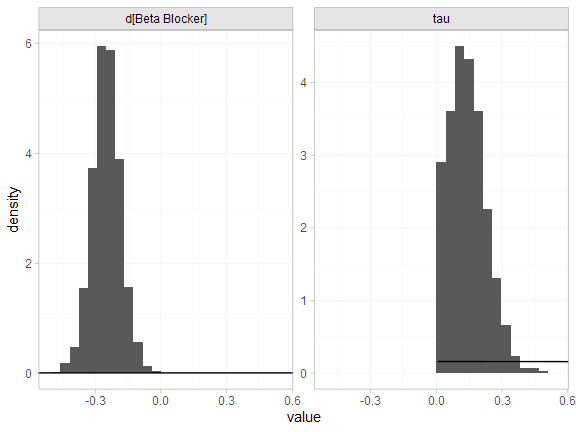#### Model comparison

Model fit can be checked using the dic() function:

(dic_FE <- dic(blocker_fit_FE))
#> Residual deviance: 46.6 (on 44 data points)
#>                pD: 23
#>               DIC: 69.6
(dic_RE <- dic(blocker_fit_RE))
#> Residual deviance: 41.6 (on 44 data points)
#>                pD: 28.6
#>               DIC: 70.2

The residual deviance is lower under the RE model, which is to be expected as this model is more flexible. However, this comes with an increased effective number of parameters (note the increase in $$p_D$$). As a result, the DIC of both models is very similar and the FE model may be preferred for parsimony.

We can also examine the residual deviance contributions with the corresponding plot() method.

plot(dic_FE)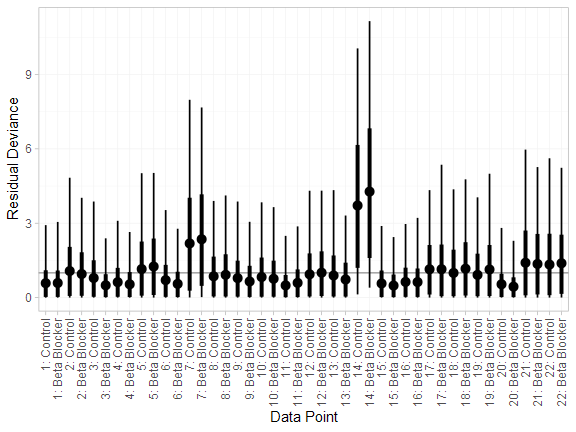plot(dic_RE)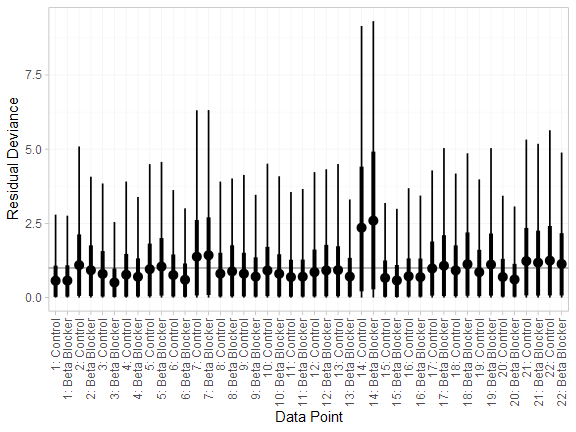There are a number of points which are not very well fit by the FE model, having posterior mean residual deviance contributions greater than 1. Study 14 is a particularly poor fit under the FE model, but its residual deviance is reduced (although still high) under the RE model. The evidence should be given further careful examination, and consideration given to other issues such as the potential for effect-modifying covariates (Dias et al. 2011).

### Further results

Dias et al. (2011) produce absolute predictions of the probability of mortality on beta blockers and control, assuming a Normal distribution on the baseline logit-probability of mortality with mean $$-2.2$$ and precision $$3.3$$. We can replicate these results using the predict() method. The baseline argument takes a distr() distribution object, with which we specify the corresponding Normal distribution. We set type = "response" to produce predicted probabilities (type = "link" would produce predicted log odds).

pred_FE <- predict(blocker_fit_FE,
baseline = distr(qnorm, mean = -2.2, sd = 3.3^-0.5),
type = "response")
pred_FE
#>                    mean   sd 2.5%  25%  50%  75% 97.5% Bulk_ESS Tail_ESS Rhat
#> pred[Control]      0.11 0.06 0.04 0.07 0.10 0.14  0.25     3810     3877    1
#> pred[Beta Blocker] 0.09 0.05 0.03 0.06 0.08 0.11  0.20     3821     3958    1
plot(pred_FE)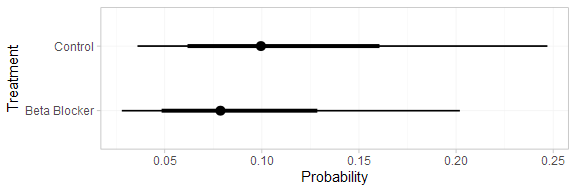pred_RE <- predict(blocker_fit_RE,
baseline = distr(qnorm, mean = -2.2, sd = 3.3^-0.5),
type = "response")
pred_RE
#>                    mean   sd 2.5%  25%  50%  75% 97.5% Bulk_ESS Tail_ESS Rhat
#> pred[Control]      0.11 0.05 0.04 0.07 0.10 0.14  0.24     3799     3975    1
#> pred[Beta Blocker] 0.09 0.05 0.03 0.06 0.08 0.11  0.20     3728     3859    1
plot(pred_RE)